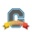# Community

cancel
Showing results for
Show  only  | Search instead for
Did you mean:New Member

## Drop lowest quiz from group (each worth different # points), weighted the rest equally by percent?

Hi,

I am an instructor in an intro astronomy course. My course has three midterm exams, which I gave as Canvas quizzes (using the old quiz module, not the new quizzes). Each quiz is worth a different number of points. In my course, I drop the lowest of these three quizzes, then average the percentages of the remaining two quizzes to get the final quiz percentage, which counts for 40% of the final grade.

Each quiz has a different number of questions and thus is worth a different number of points.

I have all my quizzes in a single group called quizzes, and I set it to drop the lowest quiz. I checked that it does in fact drop the quiz with the lowest % grade, not the one with the lowest total number of points. However, when it averages the remaining quizzes, it adds up the points from both remaining quizzes and divides by the total points possible on them, instead of adding up the percentages and dividing by 2. This effectively weights the quiz with more questions more heavily, whereas I want to weight the two remaining quizzes equally by percentage.

I read on a similar post that you can force Canvas to weight each quiz equally by putting them in separate groups, but if I do that, I'm not sure how to drop the lowest quiz.

Is there any way I can compute a group grade that is the average of percentages of each item, after dropping the lowest percentage from the group?

I'd be very grateful for any solution you might have!

Labels (1)
• ### Canvas

Tags (3)
1 SolutionCommunity Coach

Within an Assignment group the weighting is based on points.  So it's not possible to average two assignments inside of a group when their point values are not the same.

What you might consider doing is weighting your Assignment groups and set this Group weighting to 0%.  Then manually calculate the average grade and enter it in a new Assignment that gets used for actual course grading.  I know it's not ideal, but that is how I'd approach it at this point.

In the future I do have a couple of suggestions.  If you continue with Classic quizzes consider adjusting points of questions on the different quizzes so that they all equal the same amount.  Depending on the number of questions the point values can get a little weird, but I'm pretty sure I've seen people set the point values on questions to 1.67 or something so that their total equals a set value.  Warning - do not try to do this to existing quizzes with submissions.  Changing the point value on quiz questions does not trigger a regrade and it's likely to cause a mess.

If/when you move to new quizzes it will be easier.  With New Quizzes you can set the point value of the quiz independently of the sum of the points. In that case just set your Quizzes to all be the same point value, assemble your questions in each quiz to whatever you want and the overall score for the quiz will scale to the value you set it to.  This should make it much easier to do what you are trying to accomplish.

I hope that helps,

Rick

2 RepliesCommunity Coach

Within an Assignment group the weighting is based on points.  So it's not possible to average two assignments inside of a group when their point values are not the same.

What you might consider doing is weighting your Assignment groups and set this Group weighting to 0%.  Then manually calculate the average grade and enter it in a new Assignment that gets used for actual course grading.  I know it's not ideal, but that is how I'd approach it at this point.

In the future I do have a couple of suggestions.  If you continue with Classic quizzes consider adjusting points of questions on the different quizzes so that they all equal the same amount.  Depending on the number of questions the point values can get a little weird, but I'm pretty sure I've seen people set the point values on questions to 1.67 or something so that their total equals a set value.  Warning - do not try to do this to existing quizzes with submissions.  Changing the point value on quiz questions does not trigger a regrade and it's likely to cause a mess.

If/when you move to new quizzes it will be easier.  With New Quizzes you can set the point value of the quiz independently of the sum of the points. In that case just set your Quizzes to all be the same point value, assemble your questions in each quiz to whatever you want and the overall score for the quiz will scale to the value you set it to.  This should make it much easier to do what you are trying to accomplish.

I hope that helps,

RickNew Member

I think a better solution is to have the quizzes set to practice exams. Then create real exams that are each worth the same point value (say 100 points). Export the gradebook into a CSV file. Calculate the percentage of each practice exam and have it populate in the blank columns under each real exam. Then upload/import. Now the exams are each worth same point value and you can set Canvas to drop the lowest grade.

I don't see where there's an option in Quizzes to make them worth a different amount than the point value they have, especially if you create the quizes in Canvas with all of the questions/answers. Am I missing something?

Thanks,

Matt Roberts

Patrick Henry College, Purcellville, Virginia